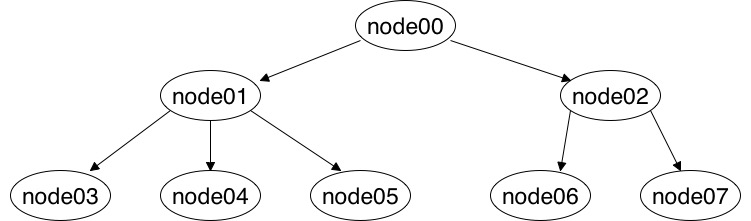# An Example of Breadth-first and Depth-first Search Algorithms in Go

Tue 20 November 2018 by jmhal

Below is an example of the two classical approaches for traversing a tree, this time written in the Go programming language.

```package main

import (
"fmt"
)

type tree struct {
value string
nodes []*tree
}

func main () {
node7 := tree{value: "node7", nodes: []*tree{}}
node6 := tree{value: "node6", nodes: []*tree{}}

node5 := tree{value: "node5", nodes: []*tree{}}
node4 := tree{value: "node4", nodes: []*tree{}}
node3 := tree{value: "node3", nodes: []*tree{}}

node1 := tree{value: "node1", nodes: []*tree{&node3, &node4, &node5}}
node2 := tree{value: "node2", nodes: []*tree{&node6, &node7}}

node0 := tree{value: "node0", nodes: []*tree{&node1, &node2}}

fmt.Println()
fmt.Println("Depth First:")
depthFirst(nodeInfo, &node0, make(map[*tree]bool))
fmt.Println()

return
}

func nodeInfo(node *tree) {
fmt.Printf(" %s ", (*node).value)
}

func breadthFirst(f func(node *tree), worklist []*tree) {
seen := make(map[*tree]bool)
for len(worklist) > 0 {
nodes := worklist
worklist = nil
for _, node := range nodes {
if !seen[node] {
f(node)
seen[node] = true
worklist = append(worklist, (*node).nodes...)
}
}
}
}

func depthFirst(f func(node *tree), node *tree, visited map[*tree]bool) {
visited[node] = true
f(node)
for _, child := range (*node). nodes {
if !visited[child] {
depthFirst(f, child, visited)
}
}
}
```

For testing the algorithms, there is a simple tree in the code. I depict the tree in the picture below:The expected output should be:

```Breadth First:
node0  node1  node2  node3  node4  node5  node6  node7
Depth First:
node0  node1  node3  node4  node5  node2  node6  node7
```

This small example shows a lot of nice features of Go. We can see that function are first order elements (nodeInfo is provided as a parameter to other functions). breadthFirst is iterative, and depthFirst is recursive. We also have a struct with pointers.